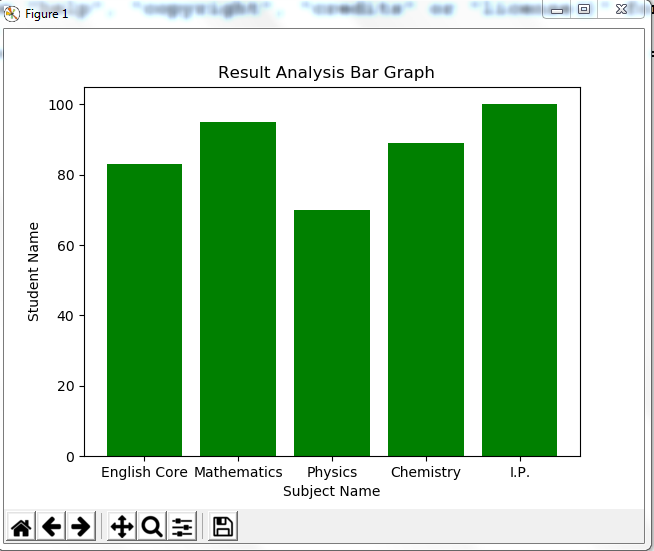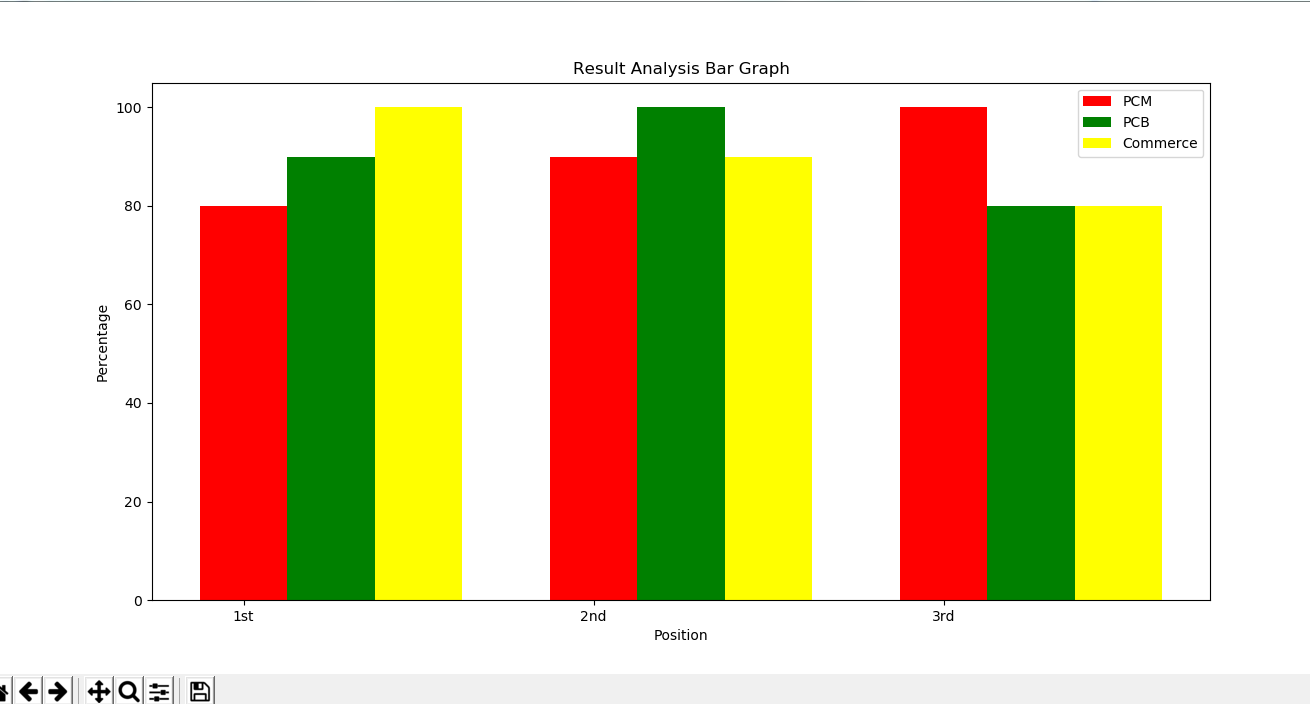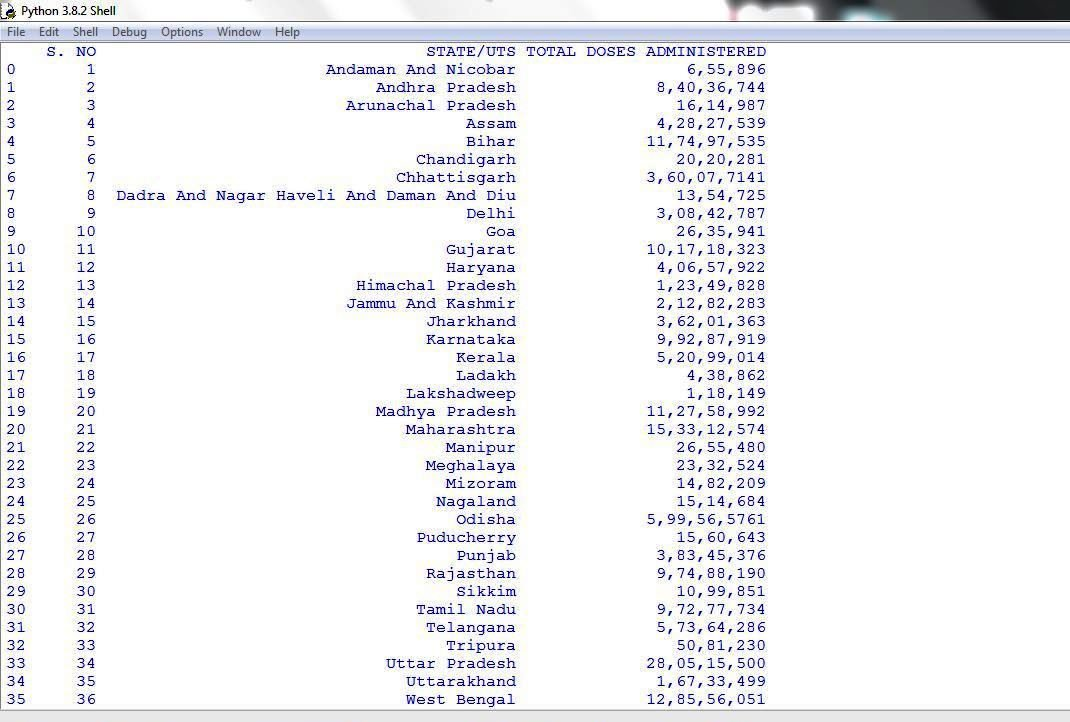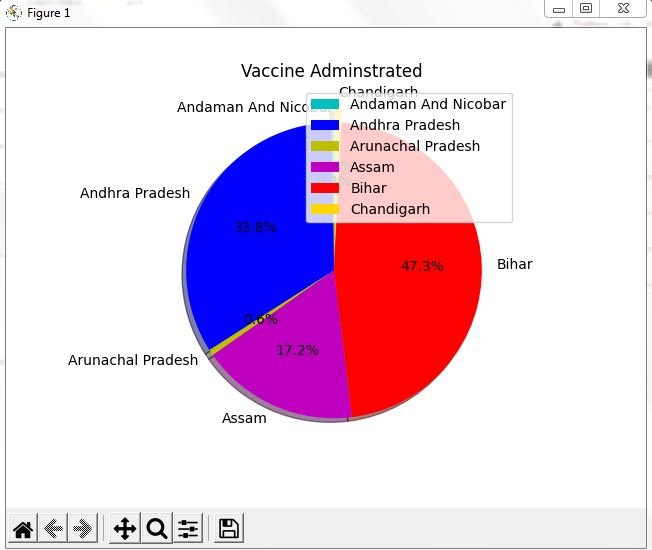# Practical File Class 12 IP Term 1

Data Handling

## Program 1: Create a panda’s series from a dictionary of values and a ndarray

```'''Python program to create a panda’s series
from a dictionary of values and a ndarray'''

import pandas as pd

import numpy as np

s=pd.Series(np.array([2,4,5,7,9,8,9]))

print(s)
```

Output:

```0 2
1 4
2 5
3 7
4 9
5 8
6 9
dtype: int32
>>>```
```# import panda lib as pd
import pandas as pd

# creating a dictionary

dictionary={'A':10, 'B':20, 'C':30}

#creating a series

series=pd.Series(dictionary)

print(series)```

Output:

```A 10
B 20
C 30
dtype: int64
>>>```

## Program 2:Given a Series, print all the elements that are above the 75th percentile.

```# import panda lib as pd
import pandas as pd
import numpy as np

series=pd.Series(np.array([1,4,6,7,8,9,11]))

print (series)

result=series.quantile(q=0.75)

print()

print ("75 percentile of the series is : ")

print(result)

print()

print ("The Elements above 75 percentile is : ")

print(series[series>result])

```

Output:

```0 1
1 4
2 6
3 7
4 8
5 9
6 11
dtype: int32

75 percentile of the series is :
8.5

The Elements above 75 percentile is :
5 9
6 11
dtype: int32
>>>```

## Program 3: Create a Data Frame quarterly sales where each row contains the item category, item name, and expenditure. Group the rows by the category and print the total expenditure per category.

```# import panda lib as pd
import pandas as pd

dictionary={'item_cat':['Tata', 'Hyundai', 'Maruti', 'Renault'],
'item_name':['Harrier', 'KIA', 'Swift', 'Duster'],
'expenditure':[2000000, 700000, 900000, 1400000]}

quarter_sales=pd.DataFrame(dictionary)

print (quarter_sales)

q_sale=quarter_sales.groupby('item_cat')

print("Result after Filtering DataFrame")

print (q_sale['item_cat','expenditure'].sum())

```

Output:

``` item_cat item_name expenditure
0 Tata Harrier 2000000
1 Hyundai KIA 700000
2 Maruti Swift 900000
3 Renault Duster 1400000
Result after Filtering DataFrame

expenditure
item_cat
Hyundai 700000
Maruti 900000
Renault 1400000
Tata 2000000
>>>```

## Program 4:Create a data frame for examination result and display row labels, column labels data types of each column and the dimensions

```import pandas as pd

dictionary={'class':['I', 'II','III','IV','V','VI','VII','VIII','IX','X','XI','XII', ],
'pass_percentage':[100,100,100,100,100,100,100,100,96,99.10,98,97.7]}

result=pd.DataFrame(dictionary)

print (result)

print (result.dtypes)

print("shape of the dataframe is : ")

print (result.shape)

```

Output:

```class pass_percentage
0 I 100.0
1 II 100.0
2 III 100.0
3 IV 100.0
4 V 100.0
5 VI 100.0
6 VII 100.0
7 VIII 100.0
8 IX 96.0
9 X 99.1
10 XI 98.0
11 XII 97.7
class object
pass_percentage float64
dtype: object
shape of the dataframe is :
(12, 2)
>>>```

## Program 5:Filter out rows based on different criteria such as duplicate rows.

```'''Python program to Filter out rows based
on different criteria such as duplicate rows'''

import pandas as pd

dic={'Name':['Ajay','Banti','Chandrkant','Deepak','Geeta','Harshit'],
'CS_Marks':[80,78,80,92,78,99]}

marks=pd.DataFrame(dic)

print(marks)

#Finding duplicate rows

print("Duplicate Rows : ")

duplicateRow = marks[marks.duplicated('CS_Marks',keep=False)]

print(duplicateRow)
```

Output:

```CS_Marks Name
0 80 Ajay
1 78 Banti
2 80 Chandrkant
3 92 Deepak
4 78 Geeta
5 99 Harshit
Duplicate Rows :
CS_Marks Name
0 80 Ajay
1 78 Banti
2 80 Chandrkant
4 78 Geeta
>>>```

## Program 6:Importing and exporting data between pandas and CSV file

```'''Importing data between pandas and CSV file'''
import pandas as pd

#make data frame

print(df)```

Output:

```ROLL No Student Name Class Marks
0 1 Arnav III 100
1 2 Bikram V 98
2 3 Charu VII 99
3 4 Dhruv VIII 91
4 5 Harshit X 80
>>>```

```'''Exporting data between pandas and CSV file'''

import pandas as pd

dic=[{'Name': 'Amit', 'Class': 12},
{'Name': 'Abhay', 'Class': 10},
{'Name': 'Harshit', 'Class': 9},
{'Name': 'Arnav', 'Class': 11}]

#make data frame

df_1=pd.DataFrame(dic)

# saving the data frame

df_1.to_csv('d:\Dataframe1.csv')```

5.2 Visualization

## Program 7:Given the school result data, analyses the performance of the students on different parameters, e.g subject wise or class wise.

```import matplotlib.pyplot as plt

subject=['English Core','Mathematics', 'Physics', 'Chemistry', 'I.P.']

percentage=[83,95,70, 89, 100]

plt.bar(subject,percentage, align='center', color='green')

plt.xlabel('Subject Name')

plt.ylabel('Student Name')

plt.title('Result Analysis Bar Graph  ')

plt.show()
```

Output:## Program 8:For the Data frames created above, analyze, and plot appropriate charts with title and legend.

```import matplotlib.pyplot as plt

import numpy as np

place=['1st','2nd','3rd']

pcm_percentage=[80,90,100]

pcb_percenatge=[90,100,80]

comm_percentage=[100,90,80]

x=np.arange(len(place))

plt.bar(x,pcm_percentage, label='PCM', width=0.25, color='red')

plt.bar(x+.25,pcb_percenatge, label='PCB', width=0.25, color='green')

plt.bar(x+.50,comm_percentage, label='Commerce', width=0.25, color='yellow')

plt.xticks(x,place)

plt.xlabel('Position')

plt.ylabel('Percentage')

plt.title('Result Analysis Bar Graph')

plt.legend()

plt.show()
```

Output:## Program 9:Take data of your interest from an open source (e.g. data.gov.in), aggregate and summarize it. Then plot it using different plotting functions of the Matplotlib library.

```import pandas as pd

import matplotlib.pyplot as plt

print(dframe)```

Output:```import pandas as pd

import matplotlib.pyplot as plt

colours=['c','b','y','m','r','gold']

exp=[0,0,0,0,0,0.1]

plt.pie(slices, labels=states, colors=colours, startangle=90, explode=exp, shadow=True, autopct='%.1f%%')